# Difference between revisions of "0708-1300/Homework Assignment 4"

Announcements go here

Read section 11 of chapter II and sections 1-3 of chapter V of Bredon's book three times:

• First time as if you were reading a novel - quickly and without too much attention to detail, just to learn what the main keywords and concepts and goals are.
• Second time like you were studying for an exam on the subject - slowly and not skipping anything, verifying every little detail.
• And then a third time, again at a quicker pace, to remind yourself of the bigger picture all those little details are there to paint.

## Doing

Solve the following problems from Bredon's book, but submit only the solutions of the problems marked with an "S":

problems on page(s)
S1, S2 100-101
S1, S2, 3 264

Also, solve and submit the following question:

Question 6.

1. Show that if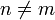$n\neq m$ then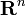${\mathbf R}^n$ is not diffeomorphic (homeomorphic via a smooth map with a smooth inverse) to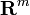${\mathbf R}^m$.
2. Show that if$n\neq m$ then${\mathbf R}^n$ is not homeomorphic to${\mathbf R}^m$.

Note that a priori the second part of this question is an order of magnitude harder than the first. I am not sure how to do it with our current techniques, though later on it will become an easy consequence of "homology theory".

## Due Date

This assignment is due in class on Tuesday November 20, 2007.

## Just for Fun

Find a geometric interpretation to the formula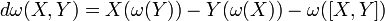$d\omega(X,Y)=X(\omega(Y))-Y(\omega(X))-\omega([X,Y])$.

(Of course, you have to first obtain a geometric understanding of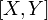$[X,Y]$, and this in itself is significant and worthwhile).

 Dror's notes above / Student's notes below

Look at the story of Barnie the polar bear. If$n\neq m$ then${\mathbf R}^n$ is 0708-1300/not homeomorphic not homeomorphic to${\mathbf R}^m$.